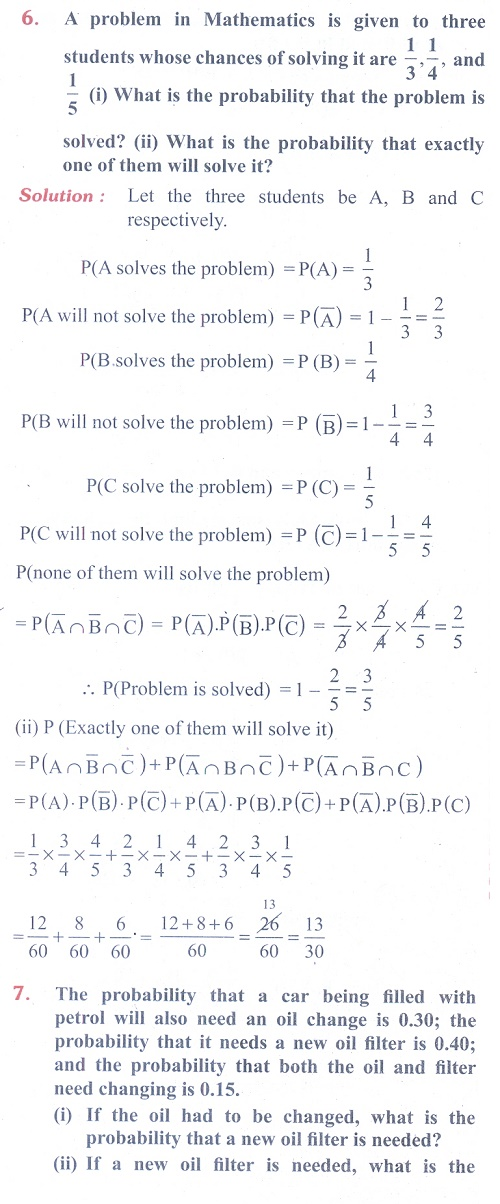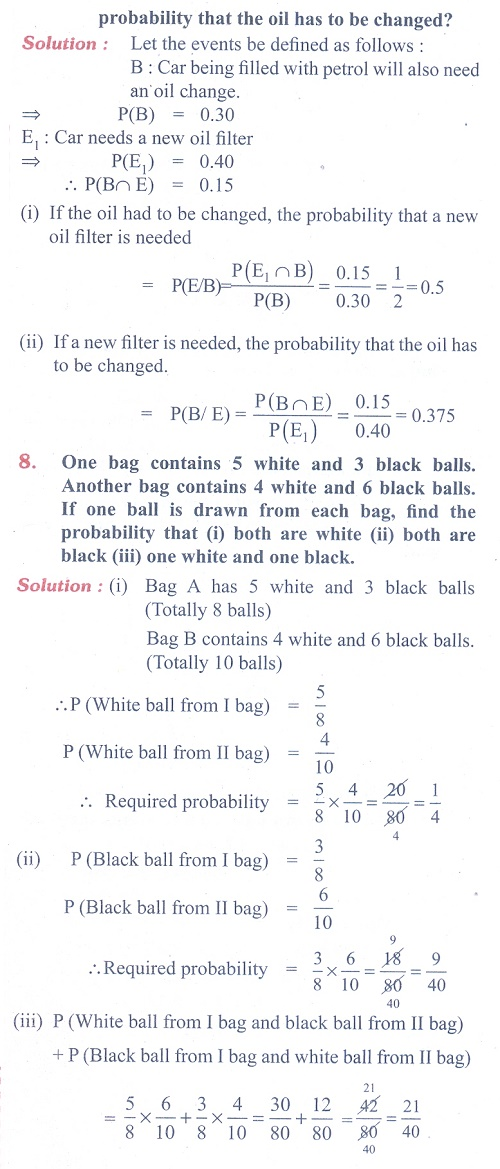Home | | Maths 11th std | Exercise 12.3: Conditional Probability

# Exercise 12.3: Conditional Probability

Maths Book back answers and solution for Exercise questions - Introduction to Probability Theory: Conditional Probability

### EXERCISE 12.3

(1) Can two events be mutually exclusive and independent simultaneously?

(2) If A and B are two events such that P (A Ōł¬ B ) = 0.7, P (A Ōł® B) = 0.2, and P(B) = 0.5, then show that A and B are independent.

(3) If A and B are two independent events such that P( A Ōł¬ B) = 0.6, P(A) = 0.2, find P(B).

(4) If P(A) =0.5, P(B) =0.8 and P(B/A) = 0.8, find P(A / B) and P( A Ōł¬ B) .

(5) If for two events A and B, P(A) 3/4 , P(B) 2/5 and AŌł¬B S (sample space), find the conditional probability P(A / B).

(6) A problem in Mathematics is given to three students whose chances of solving it are 1/3 , ┬╝ and 1/5 (i) What is the probability that the problem is solved? (ii) What is the probability that exactly one of them will solve it?

(7) The probability that a car being filled with petrol will also need an oil change is 0.30; the probability that it needs a new oil filter is 0.40; and the probability that both the oil and filter need changing is 0.15.

(i) If the oil had to be changed, what is the probability that a new oil filter is needed?

(ii) If a new oil filter is needed, what is the probability that the oil has to be changed?

(8) One bag contains 5 white and 3 black balls. Another bag contains 4 white and 6 black balls. If one ball is drawn from each bag, find the probability that (i) both are white (ii) both are black (iii) one white and one black

(9) Two thirds of students in a class are boys and rest girls. It is known that the probability of a girl getting a first grade is 0.85 and that of boys is 0.70. Find the probability that a student chosen at random will get first grade marks.

(10) Given P(A) = 0.4 and P ( A Ōł¬ B) = 0.7.  Find P(B) if

(i) A and B are mutually exclusive

(ii) A and B are independent events

(iii) P(A / B) = 0.4

(iv) P(B / A) = 0.5

(11) A year is selected at random. What is the probability that

(i) it contains 53 Sundays (ii) it is a leap year which contains 53 Sundays

(12) Suppose the chances of hitting a target by a person X is 3 times in 4 shots, by Y is 4 times in 5 shots, and by Z is 2 times in 3 shots. They fire simultaneously exactly one time. What is the probability that the target is damaged by exactly 2 hits?

Exercise 12.3 ANSWERS (1) No (3) 0.5 (4) (i) 0.5 (ii) 0.9 (5) 3/8 (6) (i) 3/5 (ii) 13/30 (7) (i) 0.5 (ii) 0.375 (8) (i) 1/4 (ii) 9/40 (iii) 21/40 (9) 0.75 (10) (i) 0.3 (ii) 0.5 (iii) 0.5 (iv) 0.5 (11) (i) 5/28 (ii) 1/14 (12) 13/30Tags : Problem Questions with Answer, Solution | Mathematics , 11th Mathematics : UNIT 12 : Introduction to Probability Theory
Study Material, Lecturing Notes, Assignment, Reference, Wiki description explanation, brief detail
11th Mathematics : UNIT 12 : Introduction to Probability Theory : Exercise 12.3: Conditional Probability | Problem Questions with Answer, Solution | Mathematics